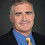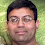## Thursday, October 20, 2022

### Alpha Tensor

In a recent post, Bill used the announcement of a new AI multiplication algorithm to discuss the applications of Strassen's famous algorithm. For this post I'd like to focus on the new algorithm itself, Alpha Tensor, the algorithm behind the algorithm, what it has actually accomplished and what it means for us theorists.

To multiply two 2x2 matrices in the usual way you need eight multiplication steps. In 1969 Strassen surprised the world by showing how to multiply those matrices using only seven multiplications.

You can recurse on larger matrices. For 4x4 matrices you can use 72=49 multiplications instead of the naïve 64. In general for nxn matrices you need roughly nlog27 ≈ n2.81 multiplications.

No one has found an algorithm for 4x4 matrices that uses less than 49 from recursing on Strassen. Alpha Tensor does so for the special case of working over GF, where addition and subtraction are interchangeable. Their algorithm does not work for general fields such as the real numbers.

Here's the full table of Alpha Tensor results from the Nature paper for multiplying a nxm matrix by a mxp matrix. The Modular column is for GF and the standard column is for general fields. Alpha tensor does improve on the best known for general fields for specific problems like multiplying a 3x4 matrix by a 4x5 matrix. Much of the press failed to make this distinction for 4x4 multiplication leading to some confusion.

What does this mean for theory? Recursing on 4x4 matrices now reduces the time for matrix multiplication to roughly n2.78 nowhere close to the best theoretical upper bound of about n2.37. The Alpha tensor result may be more practical though time will tell.

Manuel Kauers and Jakob Moosbauer shortly after Alpha Tensor announcement, reduced the 5x5 case over GF to 95 multiplications. Nice to see the last word isn't by machine (yet!) but that shouldn't reduce the excitement over Alpha Tensor. Often we see a breakthrough followed by a small improvement. Note that 95 multiplications for 5x5 matrices won't give a faster asymptotic algorithm for nxn multiplication than Strassen.

What excites me the most is not the algorithm, but the algorithm to find the algorithm. Alpha Tensor uses the tools that AlphaZero used to play Chess and Go to search the large search space of potential algorithms using Monte Carlo Tree search, basically searching at random and learning and updating the probabilities of the search. Before using machine learning, we had few good approaches to searching large combinatorial spaces of this nature.

In general, most new algorithms come from new approaches, not just from the structural decomposition we see in matrix multiplication so theorists won't be out of a job anytime soon. Nevertheless this is just another lesson that using ML has dramatically improved our ability to search through a large number of possibilities looking for a specific solution.

The Alpha Tensor Nature paper was submitted in October of 2021. A year is eternity in the ML world. I wonder what is happening now that we don't know about.

1.It is not the main point of your post, by I do not understand the following statement (read also elsewhere): "Often we see a breakthrough followed by a small improvement." For 5×5×5, AlphaTensor went from 98 multiplications to 96, and Kauers & Moosbauer from 96 to 95. I have hard time considering the first as a "breakthrough" and the second as a "small improvement". Especially since it becomes harder and harder to reduce the number of multiplications… Also one can have a look at Kauers & Moosbauer technique: This is an clever computer search. Ok this does not use the trendy Deep Learning technology, but both are of the same nature!

I am not saying that Alpha Tensor result is uninteresting of course. But I wonder (not really…) why there is so much buzz around it, while concurrent approaches that are not worse, nor older or more classical, are overlooked. Otherwise stated, there are a lot of exciting new techniques to produce algorithms, and Deep Learning is merely one of them.

1.I didn't mean to diminish the work of Kauers and Mossbauer but would they have even attempted their work if it wasn't for AlphaTensor showing such improvements are possible?

2.The excitement (or fear) about Deep Learning is because it is a general purpose method that can in principle work for many problems without human intervention/creativity.

3.Isn't Monte Carlo algorithm considered/classified as a probabilistic algorithm; ie, not machine learning?or both?
(Sorry if my comment doesn't make sense for you, I have once studied a course on probabilistic algorithms and I do remember Monte Carlo algorithms but never worked machine learning)

1.Monte Carlo is a term generally used for randomized algorithms. Monte Carlo Tree Search is a method for searching game trees by choosing random moves, that surprising produced some of the first decent Go program. AlphaGo honed the MCTS process using deep learning to beat the best humans.

4.MC tree search is a wonderful technique for sure. But IMO the magic is still by Strassen back in '69, to come up with his idea of using 7 mults for 2x2 - what's in those 7 terms, and how they combine in two ways to produce the two left diagonal and the two right diagonal terms, is genius!!